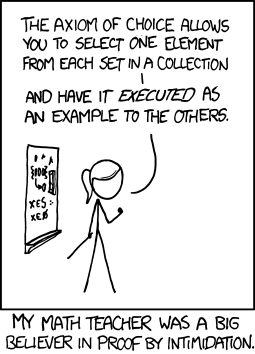Axiom Of Choice - The Programmer's Guide
Written by Mike James
Thursday, 29 March 2018
Article Index
Axiom Of Choice - The Programmer's Guide

Many mathematicians seem to satisfy themselves with a vague (and sometimes precise) idea that in situations like this the choice function could somehow be an arbitrary "pick one point of each set"  - it doesn't matter what it is, just pick one. You can see that in simple cases the choice function is doing something we might be able to name - the mid-point, the smallest value, etc, but this new arbitrary choice function is more like a random selection.

Bertrand Russell tried to explain this idea by the example of socks and shoes. If you have an infinite collection of pairs of shoes then the choice function could be either "pick the right shoe" or "pick the left shoe" - no need for the axiom of choice. Now consider a similar collection of pairs of socks. There is no obvious choice function because there is no way to distinguish between the socks making up the pair. In this case the axiom of choice has to be invoked to say that you can indeed pick a sock from each pair.

The sock example sounds reasonable, but is an arbitrary choice really a computable function? In the case of socks it seems reasonable, but in the case of all sub-sets of the reals it isn't as obvious.

There are also so many deep resonances here with other subjects. Do the pairs of shoes and socks remind you of the behavior of fermions and bosons? If you are a physicist, they do. The inability to provide a computable function without using a random choice in the case of the socks says something about algorithmic information, but what exactly isn't obvious and so on.

You can now also see why the axiom of choice comes into the proof of Zermelo's well-ordering theorem. If you can find a function that is irregular enough to pick an arbitrary point in each set then presumably there is no reason to be able to find a function that orders each set. In fact the two ideas, the axiom of choice and the well-ordering theorem are equivalent.

## Non-Constructive

In mathematics the axiom of choice is usually invoked to suppose that there is a choice function without having to explicitly supply one - and this leads to conclusions that might not undisputed paradoxes but can be very troubling. The problem is that just insisting that a procedure can be done without explaining how it can be done isn't constructive. It leads to mathematics with objects and procedures which provably exist without giving the exact algorithm to create such an object.

For example, the best known is the Banach-Tarski paradox which says that if you take a 3D solid unit sphere you can "carve it up" into a finite number of pieces and then using rotations and translations reassemble it into two identical 3D solid unit spheres. We seem to have created twice the volume of stuff without actually creating anything at all. The pieces are constructed using the axiom of choice but obviously without giving the choice function used to do the job.

If you think that doubling the volume of stuff is simply a crazy illogical idea then you are forgetting some of the strange properties of infinity. Take an infinite set of the integers and split it into a set or even and a set of odd integers - both sets are also infinite. So you start with an infinite set and split it in two and you have two infinite sets - perfectly logical. In the case of the Banach-Tarski paradox the sets aren't of infinite extent i.e they are bounded but they are continuous and hence have aleph one points. In the same way as the countable sets can be split into two parts each infinite so the ball can be split into two parts each with aleph one points inside. However to do this you have to be able to select an aleph one number of points from an aleph one number of sets i.e. a choice function must exist.

If you think that this is just silly and proof that the axiom of choice isn't valid, then you need to know just how many results in less controversial mathematics depend on it.

For example, every Hilbert space has an orthonormal basic, the Hahn-Banach theorem, the additive groups on R and C are isomorphic, the theorem that every countable union of countable sets is countable and so on... they are all fairly basic properties that we expect to be true.

At the end of the day the axiom of choice is more illuminating about the properties of infinite sets than anything else. Mathematics makes use of it but there is a certain caution about theorems that rely on it.

xkcd's math teacher can rest assured that as long as the sets are restricted to a finite collection then it is always possible to pick an element for execution - but when we get to infinite collections then you have to enforce the axiom of choice first.More cartoon fun at xkcd a webcomic of romance,sarcasm, math, and language

Perhaps it is the axiom of choice that should be executed.

## A Programmers Guide To Theory - NP & Co-NP#### Contents

*To be revised